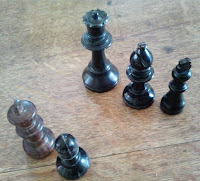## 9 Mar 2013

### Help Kids Add and Subtract Numbers Up to Ten

My daughter needed help with adding and subtracting numbers up to ten. This is what we did, based on suggestions from her class teacher.
Ingredients
Ten chess pieces: five black, five white
Coloured felt pens
Exercise book'Well done' stickers
Total Time: 40 minutes

1. Set out five chess pieces and put two on one side. Ask 'how many do I have' if I add 'one', 'two' then 'three'. Then set out some sums - 2+1=, 2+2=, 2+3= in the exercise book.

What next?

2. Set out five black pieces in a row. 'Take away' one, then two, then three etc, asking each time, 'how many do I have?' Then set these out as sums, making sure to explain what the minus or subtract sign means.

3. Now, put a row of five white next to the row of black. Repeat the exercises in a similar pattern for adding up to ten or less, and subtracting from ten.
5 + 1 =        10 - 1 =
5 + 2 =        10 - 2 =
5 + 3 =        10 - 3 =
5 + 4 =        10 - 4 =
5 + 5 =        10 - 5 =
etc

4. When we'd done, her book looked like this:

We made a number spider (above right) 1+9 = 2+8 = 3+7 = 4+6 = 5+5 = 10. Then we drew the pairs of numbers that add up to ten (as shown below) and my daughter coloured it in.